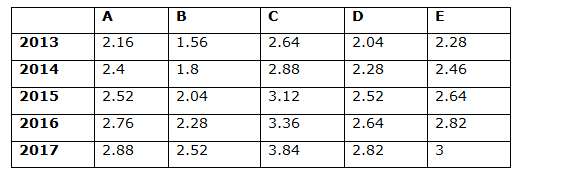# CWC/FCI Prelims 2019 – Quantitative Aptitude Questions (Day-56)

Dear Readers, Exam Race for the Year 2019 has already started, CWC/ FCI will be the most expected exam among the aspirants. Preparation and performance both requisite to clear CWC/FCI Exam. To enrich your preparation here we are providing a new series of Practice Questions on CWC/ FCI Quantitative Aptitude Section. Aspirants, practice these questions on a regular basis to improve your score in the aptitude section. Start your effective preparation from the right beginning to get success in the upcoming CWC/FCI Exam.

[WpProQuiz 6218]

Directions (Q. 1 – 5): In the following questions, two equations I and II are given. You have to solve both the equations and give answer as,

a) If x > y

b) If x ≥ y

c) If x < y

d) If x ≤ y

e) If x = y or the relation cannot be established

1) I) x2 + 18x + 72 = 0

II) y2 + 30 y + 56 = 0

2) I) 2x2 – 9x + 4 = 0

II) y2 – 14y + 40 = 0

3) I) 2x – 5y = 12

II) 5x – 2y = -12

4) I) x2 – 8x – 48 = 0

II) y2 + 10y + 24 = 0

5) I) 5x2 + 29x + 42 = 0

II) 4y2 + 23y + 33 = 0

Directions (Q. 6 – 10): Study the following information carefully and answer the given questions.

The following table shows the annual salary (In lakhs) of 5 different persons over the years.6) Find the difference between the total annual salary in the year 2013 to that of 2015 of all the given persons together?

a) 2.16 lakhs

b) 2.52 lakhs

c) 2.34 lakhs

d) 2.78 lakhs

e) None of these

7) Find the ratio between the annual salary of A to that of E of all the given years together?

a) 15: 19

b) 27: 31

c) 64: 77

d) 53: 55

e) None of these

8) Find the average annual salary of B of all the given years together?

a) 2.56 lakhs

b) 1.72 lakhs

c) 2.04 lakhs

d) 2.28 lakhs

e) None of these

9) The total annual salary of B, C and D together in the year 2014 is approximately what percentage of total annual salary of A, C and E together in the year 2016?

a) 92 %

b) 78 %

c) 64 %

d) 106 %

e) 115 %

10) Find the difference between the average annual salaries of A to that of D of all the given years together?

a) Rs. 42600

b) Rs. 40800

c) Rs. 36200

d) Rs. 38400

e) None of these

I) x2 +18x + 72 = 0

(x + 12) (x + 6) = 0

x = -12, -6

II) y2 + 30 y + 56 = 0

(y + 28)(y + 2) = 0

y = -28, -2

Can’t be determined

I) 2x2 – 9x + 4 = 0

2x2 – x – 8x + 4 = 0

x (2x – 1) – 4 (2x – 1) = 0

(x – 4) (2x – 1) = 0

x = 4, 1/2

II) y2 – 14y + 40 = 0

(y – 10) (y – 4) = 0

y = 10, 4

x ≤ y

2x – 5y = 12 –> (1)

5x – 2y = -12 —> (2)

By substituting (1) and (2), we get,

x = -4, y = -4

x = y

I) x2 – 8x – 48 = 0

(x – 12) (x + 4) = 0

x = 12, -4

II) y2 + 10y + 24 = 0

(y + 6) (y + 4) = 0

y = -6, -4

x ≥ y

I) 5x2 + 29x + 42 = 0

5x2 + 15x + 14x + 42 = 0

5x(x + 3) + 14 (x + 3) = 0

(5x + 14) (x + 3) = 0

x = -14/5, -3 = -2.8, -3

II) 4y2 + 23y + 33 = 0

4y2 + 12y + 11y + 33 = 0

4y(y + 3) + 11 (y + 3) = 0

(4y + 11) (y + 3) = 0

y = -11/4, -3 = -2.75, -3

Can’t be determined

The total annual salary in the year 2013 of all the given persons together

= > 2.16 + 1.56 + 2.64 + 2.04 + 2.28 = 10.68 lakhs

The total annual salary in the year 2015 of all the given persons together

= > 2.52 + 2.04 + 3.12 + 2.52 + 2.64 = 12.84 lakhs

Required difference = 2.16 lakhs

The annual salary of A of all the given years together

= > 2.16 + 2.4 + 2.52 + 2.76 + 2.88 = 12.72 lakhs

The annual salary of E of all the given years together

= > 2.28 + 2.46 + 2.64 + 2.82 + 3 = 13.2 lakhs

Required ratio = 12.72: 13.2 = 53: 55

The average annual salary of B of all the given years together

= > (1.56 + 1.8 + 2.04 + 2.28 + 2.52)/5

= > 2.04 lakhs

The total annual salary of B, C and D together in the year 2014

= > 1.8 + 2.88 + 2.28 = 6.96 lakhs

The total annual salary of A, C and E together in the year 2016

= > 2.76 + 3.36 + 2.82 = 8.94 lakhs

Required % = (6.96/8.94)*100 = 77.85 % = 78 %

The average annual salaries of A of all the given years together

= > (2.16 + 2.4 + 2.52 + 2.76 + 2.88)/5 = 2.544 lakhs

The average annual salaries of D of all the given years together

= > (2.04 + 2.28 + 3.12 + 3.36 + 3.84)/5 = 2.928 lakhs

Required difference = 2.928 – 2.544 = 0.384 lakhs = Rs. 38400

Related Articles: